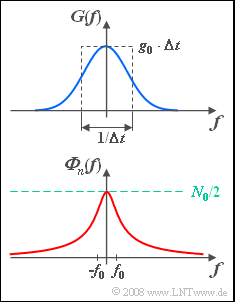# Exercise 5.8: Matched Filter for Colored InterferenceSpectrum  $G(f)\ \bullet\!\!\!-\!\!\!-\!\!\!-\!\!\circ\, \ g(t)$;
PSD ${\it \Phi}_n (f)$  of the interference

At the input of a filter there is a Gaussian pulse

$$g(t) = g_0 \cdot {\rm{e}}^{ - {\rm{\pi }}\left( {t/\Delta t} \right)^2 }$$

with amplitude  $g_0 = 2 \hspace{0.08cm}\rm V$  and equivalent pulse duration  $\Delta t = 1 \hspace{0.08cm}\rm ms$.

• The corresponding spectral function  $G(f)$  is sketched above.
• The energy of this Gaussian pulse is given as follows:
$$E_g = \int_{ - \infty }^{ + \infty } {g^2(t) \ {\rm{d}}t = \frac{g_0 ^2 \cdot \Delta t}{\sqrt 2 }} = 2.83 \cdot 10^{ - 3} \;{\rm{V}}^{\rm{2}} {\rm{s}}.$$

An interference  $n(t)$  is superimposed on the impulse  $g(t)$,  which largely covers the impulse.

Two alternatives are considered for this purpose:

• Let the bilateral interference power density be constant  (only for the first subtask):
$${\it \Phi}_n (f) = \frac{N_0 }{2},\quad N_0 = 10^{ - 6} \;{\rm{V}}^{\rm{2}}/ {\rm{Hz}}.$$
• Let the interference signal  $n(t)$  be colored with the following interference power-spectral density  $\rm (PSD)$:
$${\it \Phi}_n (f) = \frac{N_0 /2}{{1 + \left( {f/f_0 } \right)^2 }},\quad f_0 = 500\;{\rm{Hz}}.$$

This second PSD response can be modeled,  for example,  from white noise by a shape filter with frequency response  (first order low-pass):

$$H_{\rm N}(f) = \frac{1}{{1 + {\rm{j}}\cdot f/f_0 }}\quad\bullet\!\!\!-\!\!\!-\!\!\!-\!\!\circ\, \quad h_{\rm N}(t) = 2{\rm{\pi }}f_0 \cdot {\rm{e}}^{ - 2{\rm{\pi }}f_0 t}$$

Further,  let it hold:

• Let the filter be optimally matched to the transmitted pulse shape  $g(t)$  and the interference power-spectral density  ${\it \Phi}_n (f)$,  respectively:
$$H(f) = H_{\rm MF}(f).$$
• The filter constant  $K_{\rm MF}$  is to be chosen such that  $H(f= 0) =1$.
• Let the detection time be  $T_{\rm D}= 0$  for simplicity  (acausal system description).

Notes:

$$\frac{1}{{\sqrt {2{\rm{\pi }}} }}\int_{ - \infty }^{ + \infty } {x^2 \cdot {\rm e}^{ - x^2 /2} \,\,{\rm{d}}x} = 1.$$

### Questions

1

What is the  "maximum signal-to-interference ratio"   $\rho_{\text{MF, WN}}$  (in dB)  in the white noise case?

 $10 \cdot \lg \hspace{0.1cm} \rho_{\text{MF, WN}} \ = \$ $\ \rm dB$

2

Calculate the matched filter frequency response in the presence of colored interference.
What is the magnitude of  $H_\text{MF}(f)$  at frequency  $f = 1 \hspace{0.08cm} \rm kHz$?

 $|H_\text{MF}(f = 1 \hspace{0.08cm} \rm kHz)| \ = \$

3

What is the  "maximum signal-to-interference ratio"   $\rho_{\text{MF}}$  at the receiver in the case of the given colored interference?  Give reasons for the better result.

 $10 \cdot \lg \hspace{0.1cm} \rho_{\text{MF}} \ = \$ $\ \rm dB$

### Solution

#### Solution

(1)  For white noise,  according to the general equations in the theory section:

$$\rho_{d,\ \rm WR} = \frac{2E_g }{N_0 } = \frac{{2 \cdot 2.83 \cdot 10^{ - 3} \;{\rm{V}}^{\rm{2}} {\rm{s}}}}{{10^{ - 6} \;{\rm{V}}^{\rm{2}}/ {\rm{Hz}}}} = 5.66 \cdot 10^3 \quad \Rightarrow \quad 10\lg \cdot \rho _{d,\ \rm WR} \hspace{0.15cm}\underline {= 37.53\;{\rm{dB}}}.$$

(2)  For the frequency response in the case of colored interferences,  under the condition  $T_{\rm D}= 0$:

$$H_\text{MF} (f) = K_\text{MF}\cdot \frac{G^{\star} (f)}{\left| {H_{\rm N} (f)} \right|^2 }\hspace{0.2cm}{\rm with}\hspace{0.15cm} G(f) = g_0 \cdot \Delta t \cdot {\rm{e}}^{ - {\rm{\pi }}\left( {\Delta t \hspace{0.03cm}\cdot \hspace{0.03cm}f} \right)^2 } ,\hspace{0.15cm}\frac{1}{\left| {H_{\rm N} (f)} \right|^2 } =1+\left( f/f_0 \right)^2.$$
• From the condition  $H_\text{MF}(f = 0) = 1$  it follows  $K_\text{MF} = 1/(g_0 \cdot \Delta t)$.  Thus one obtains:
$$H_{\rm MF} (f) = {\rm{e}}^{ - {\rm{\pi }}[ {\Delta t \cdot f} ]^2 } \cdot \left( {1 + \left(f/f_0 \right)^2 } \right).$$
• For white  (frequency-independent)  noise,  the matched filter would be given by the first term alone,  which causes the matching to the pulse  $g(t)$.
• For colored interference  ⇒  PSD  ${\it \Phi}_n(f)$,  higher frequencies are raised by the correction term  $1+\left( f/f_0 \right)^2$  because in this range the interference is lower.
• For  $f = 1/\Delta t = 2f_0 = 1\hspace{0.08cm} \rm kHz$  we obtain:
$$H_{\rm MF} ( {f = {1}/{\Delta t}} ) = {\rm{e}}^{ - {\rm{\pi }}} \cdot \left( {1 + 2^2 } \right) \hspace{0.15cm}\underline {= 0.216}.$$

(3)  In general,  the S/N ratio at the output of the matched filter is:

$$\rho _d = \int_{ - \infty }^{ + \infty } {\frac{\left| {G(f)} \right|^2 }{\it{\Phi _n} \left( f \right)}\,\,{\rm{d}}f = } \int_{ - \infty }^{ + \infty } {\frac{\left| {G(f)} \right|^2 }{N_0 /2}} \, \,{\rm{d}}f \hspace{0.3cm}+ \hspace{0.3cm} \int_{ - \infty }^{ + \infty } {\frac{\left| {G(f)} \right|^2 }{N_0 /2}} \cdot \frac{f^2 }{f_0 ^2 }\,\,{\rm{d}}f.$$
• The first summand is equal to the S/N ratio withn white noise.  For the second summand,  we obtain:
$$\Delta \rho _d = \frac{g_0 ^2 \cdot \Delta t^2 }{N_0 /2 \cdot f_0 ^2 }\cdot \int_{ - \infty }^{ + \infty } {f^2 \cdot {\rm{e}}^{ - 2{\rm{\pi }}\left( {f \cdot \Delta t} \right)^2 } }\,\, {\rm{d}}f.$$
• After substituting  $x = 2 \cdot \pi^{0.5}\cdot f \cdot \Delta t$,  this integral becomes:
$$\Delta \rho _d = \frac{\sqrt 2 \cdot g_0 ^2 \cdot \Delta t}{N_0 } \cdot \frac{1}{{4{\rm{\pi }}\left( {\Delta t \cdot f_0 } \right)^2 }} \cdot \int_{ - \infty }^{ + \infty } {\frac{x^2 }{\sqrt {2{\rm{\pi }}} }} \cdot {\rm{e}}^{ - x^2 /2}\,\, {\rm{d}}x.$$
• This particular integral was given in the front;  it has the value  $1$.  The first factor again describes the S/N ratio with white noise.
• This gives the following equations:
$$\Delta \rho _d = \rho _{d,\rm WR} \cdot \frac{1}{{4{\rm{\pi }}\left( {\Delta t \cdot f_0 } \right)^2 }}, \hspace{1cm} \rho _d = \rho _{d,\rm WR} + \Delta \rho _d = \rho _{d, \rm WR} \left( {1 + \frac{1}{{4{\rm{\pi }}\left( {\Delta t \cdot f_0 } \right)^2 }}} \right).$$
$$\Rightarrow \hspace{0.3cm} \Delta t \cdot f_0 = 0.5 : \hspace{0.3cm} \rho _d = 1.318 \cdot \rho _{d,\rm WR} = 7.46 \cdot 10^3 \hspace{0.3cm} \Rightarrow \quad 10\lg \rho _d \hspace{0.15cm}\underline {= 38.73\;{\rm{dB}}}.$$

Conclusion: There is a  $1.2 \; \rm dB$  better result than with white noise,  because  ${\it \Phi}_n(f)$  is here smaller than  $N_0/2$ in the whole frequency range except for the frequency  $f = 0$  $($here the equal sign applies$)$.  This fact is also used by the matched filter.